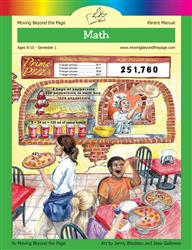Age 5-7 Reading Is Now Available!

# Common Core Alignment

CCSS.Math.Content.4.NBT.5 - Multiply a whole number of up to four digits by a one-digit whole number, and multiply two two-digit numbers, using strategies based on place value and the properties of operations. Illustrate and explain the calculation by using equations, rectangular arrays, and/or area models.

## 5: MathUnit 4: Multi-Digit Multiplication
Lesson 2: Multiples of 10, 100, and 1000
Lesson 3: Multiples of 10, 100, and Beyond!
Lesson 4: Multi-Digit Multiplication Using Arrays
Lesson 5: The Area Model
Lesson 6: The Standard Multiplication Algorithm
Lesson 7: Multiplication Practice
Lesson 8: Unit Test
Final Project: Multiplication Contract